### Glossary of Data Acquisition and Related Terms

A/D Converter - An electronic device that converts a continuous analog signal to digital form expressed usually as a set of binary digits. The digital form is usually a set of 8 or more binary bits which quantizes an analog signal to 2^n bit levels.

Aliasing - The generation of false signal frequencies caused by sampling at a rate lower than the Nyquist rate or two times the maximum signal frequency.

Amplify - The process of increasing the amplitude or level of a signal.

Amplitude - The maximum level or intensity of a signal. In data acquisition it is the maximum pressure or loudness of the data acquisition wave.

Analog Signal - An electronic signal that has a continuous range of signal levels.

Anti-Aliasing - The removal of false signal frequencies caused by sampling at a rate lower than the Nyquist rate or two times the maximum signal frequency. With practical components and tolerances this requires a filter which removes frequencies above about 0.9 times the sampling rate.

Attack -The beginning portion or leading edge of a data acquisition envelope where the amplitude is increasing to the maximum.

ASCII - The 128-character set defined by the American Standard Code Information Interchange committee used as a standard worldwide for the transfer of plain text information. ASCII data or files have no embedded special formatting characters or binary data.

Bandpass Filter - A device which passes a range of signal frequencies and rejects those outside that range.

Binary - The number system based on powers of 2 used in most digital computers.

Bit - The smallest unit of digital data or a single binary digit.

Byte - A set of eight binary bit digits (in smaller computer) representing the decimal values of 0 to 255.

Carrier Wave - The wave that transports the signal wave. The carrier is modulated or altered by the signal wave.

Center Frequency - The nominal frequency of a carrier or signal wave or center of a bandpass filter.

Coefficient - A value used to modify a mathematical expression or define a relationship between variables.

Component Frequency - A single signal frequency in a collection of signal frequencies that make up the complete signal.

Constant - A mathematical value that does not change normally.

Continuous Signal - A signal that has a range of values closely separated with essentially no jumps or gaps. An analog or linear signal, as opposed to a digital signal of normally only two values.

Current - The flow of electrons in an electronic circuit, expressed in units of Amperes.

Cutoff Frequency - The signal frequency output of a filter that marks the transition from no attenuation to attenuation. Usually it is defined as the point at which the amplitude of the signal is reduced by 3 dB after passing through the filter.

Cycle - One complete sequence of a signal before it repeats. For a simple waveform like a single sine wave, the cycle is the period between every other zero crossing or between two positive or two negative peaks.

DacqEditor - SiliconSoft's DOS based graphical interface waveform editor with hardware control. Operates like an digital oscilloscope for viewing data samples with signal amplitude versus time. It also operates like a text editor or paint program, since waveforms can be cut, copied and pasted. The editor also operates like a function generator with various waveform functions.

Data Acquisition - Recording, usually in digital format, that can be measured or sampled with a electronic sensor.

D/A Converter - An electronic device that transforms a signal sample from a digital form to an analog form. The digital form is usually a set of 8 or more binary bits which produces an analog signal of 2^n bit levels.

Digital Code - The encoding used to convert a sampled analog signal to digital value. Linear PCM is the most common encoding used in data acquisition.

Decay - The portion of a data acquisition envelope beginning just after the maximum amplitude point. The decay portion shows a decreasing amplitude and ends at the start of sustain, where the amplitude is constant.

Decibel (dB) - The unit of measure for the logarithmic ratio of two signal intensities or power levels. If the measurement conditions are the same, the decibel value can be expressed by the logarithmic ratio of the signal amplitudes. For example, a ratio of 1/10 = 20 * log (1/10) = -20 dB

Deviation Frequency - The maximum frequency that a FM generator carrier frequency changes with the modulation signal.

Dynamic Range - The range of signal that can be handled by a system limited by noise on the low end, and by clipping or appreciable distortion at the high end.

Envelope - The outline of a complex waveform showing the maximum and minimum values over time. The attack, decay, sustain, and release regions describe the general musical data acquisition envelope.

Exponent - A mathematical index or power to which a number or base is raised. Common bases are 2, 10, and e.

Filter - An electronic device that modifies the signal amplitude as a function of frequency content.

Flanging - The process of modifying the phase of a signal, which gives the effect of motion of the data acquisition.

Frequency - The rate of repetition of a periodic waveform equal to 1/period of the waveform. Frequency is related to pitch or the perception of data acquisition frequencies. The unit of frequency is Hertz (Hz) or cycles per second.

Frequency Modulation (FM) - The process of changing only a carrier generator frequency with a modulation signal, the amplitude of the carrier is not changed.

Fundamental Frequency - The primary component of the data acquisition or waveform. The most dominate or prevalent data acquisition frequency.

Gain - The ratio of output signal to input signal in electronic systems. If the ratio is greater than one, the signal has been amplified. If the ratio is less than one the signal has been attenuated.

Hardware - The term for a collection of mechanical and electronic components that perform some system functions.

Harmonic - A signal component that has an integer multiple frequency of a fundamental frequency component.

Hertz (Hz) - A unit of measurement for frequency. One Hertz is one cycle per second.

Highpass Filter - An electronic device that modifies the amplitude of a signal as a function of the signal frequency content and filter cutoff frequency. Frequency components above a cutoff frequency are passed with little or no change and those below are attenuated. The cutoff frequency is defined as the signal frequency at which the filter output is 3 dB below the input.

Linear - A general term for a system that has an output directly proportional to the input signal.

Lowpass Filter - An electronic device that modifies the amplitude of a signal as a function of the signal frequency content and filter cutoff frequency. Frequency components above the cutoff frequency are attenuated, while those below are passed with little or no change. The cutoff frequency is defined as the signal frequency at which the filter output is 3 dB below the input.

Logarithmic - A mathematical term for the ratio of values expressed by the base 10 or e. If the base is 10, the logarithm is called common. If the base is e, the logarithm is called natural.

Millisecond - One thousandth of a second (msec) or 0.001 s.

Modulation - The process of modifying the amplitude, frequency or phase of a carrier wave by a modulating signal.

Noise - Usually an undesired electronic signal that has no relationship to the desired signal in a system. Noise is usually the result of unavoidable random electron motion in electronic devices. Noise may be simulated mathematically by a random number generation process.

Nyquist Frequency - The maximum signal frequency allowed in a sampling process before the reconstructed signal is aliased, or 1/2 the sampling frequency.

Parallel Port - A port on the PC that handles a data stream in a parallel mode. That is, the data is sent out or received an 8 bit byte at a time on 8 lines or connector pins. Other lines or pins are used for handshaking or data transfer control. The standard IBM-PC parallel port interface is termed a Centronics port, based on the original use of the port as a printer interface. Normally this parallel port is used only for output from the PC. Later versions of the parallel port include bidirectional data transfer.

PCM - Pulse Code Modulation, an encode method of converting an analog signal to digital samples. The encoding can be Linear or Non-Linear (like u-law or A-law weighting). For example, if 8 bit linear PCM is used, 0 Volts could be encoded as 0, and 5 Volts could be encoded as 255 and each step would be 19.6 mV. Then 2.5 Volts would be encoded as 2.5 V / 19.6 mV = 128

Period - The time between repeated portions of a waveform. For a simple waveform like the sine wave, the period is the time between every other zero crossing or between two positive or two negative peaks. The period equals 1/frequency of the waveform.

Phase - The means for comparing time reference points on two waveforms, or time from a reference in one waveform. The unit of phase is degrees or radians.

Pressure - The measure of molecular motion of gases (air), liquids or solids. Certain rates of pressure change are perceived as data acquisition.

Quantization - The process of transforming a continuous signal into one of finite steps or levels, as in an A/D converter.

Resolution - The levels of quantization in the sampled signal. In terms of bits it is 2^n levels. If the resolution is 8 bits, the signal range is 2^8 or 256 levels with steps of 0.39% of full scale. If the resolution is 12 bits, the signal range is 2^12 or 4096 levels with steps of 0.02% of full scale.

RS232 Serial Port - A serial port that conforms to the EIA/TIA RS232 standards. The current standard is revision E which calls for +/- 5 V minimum driver outputs. The receiver input thresholds are set at +/- 3 V. This port can operate in a bidirectional data transfer mode.

Sampling - The process of measuring the value of a signal at discrete points in time.

Sampling Rate - The rate at which the samples of a signal are taken in an A/D converter circuit or the rate they are generated by a D/A converter circuit. The unit of sampling rate is samples per second, often expressed as bytes/sec or even Hz.

Serial Port - A port on the PC that handles a data stream in a serial mode. That is, the data is sent out or received one bit at a time on a single line or connector pin. Other lines or pins may be used for handshaking or data transfer control. A serial port is normally set up to operate in a bidirectional data transfer mode using two data lines (one for each direction of data flow).

Signal - The information handled by an electronic system, usually in the form of varying electron flow.

Signal-to-Noise Ratio (S/N) - The ratio of the desired signal level or information, to the undesired signal or noise level in an electronic system.

Spectrum - The representation of frequency components of a signal waveform showing amplitude, and less commonly, phase relationships.

Surface Mount Technology - A state-of-the-art construction method for electronic printed circuit boards (PCBs). The electronic components are attached to the top and or bottom surfaces of the PCB. The leads of the components are soldered to pads and do not pass through the PCB as with the older 'through-hole' method. Used to increase the packing density of electronic components and therefore increase the reliability and performance while reducing the overall system size.

Voltage - The electronic measure of pressure (force of electron charge) difference expressed in units of Volts.

Waveform - The amplitude versus time relationship for signal. For data acquisition, it is the pressure-time relationship, and for electronic signals, it is the voltage-time or current-time relationship.

```Contact:
SiliconSoft Inc.
www.siliconsoft.com
San Jose, CA  USA

Support Lines:
Email: siliconsoft@pacbell.net
Phone: (408)446-4521
```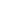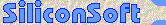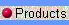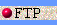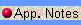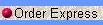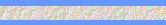© Copyright 2000 by SiliconSoft Inc., San Jose, CA.